﻿ 基于接近觉的机械臂避障路径规划
 机器人2022, Vol. 44Issue (5): 601-6120

LI Long, CHEN Hewei, WANG Tianhong, ZHANG Quan, WANG Guopeng, TIAN Yingzhong, PENG Yan, LUO Jun. Obstacle Avoidance Path Planning of Manipulator Based on Proximity[J]. ROBOT, 2022, 44(5): 601-612.1. 上海大学机电工程与自动化学院, 上海 200444;
2. 上海市智能制造及机器人重点实验室, 上海 200444;
3. 上海大学人工智能研究院, 上海 200444;
4. 江苏省先进机器人技术重点实验室, 江苏 苏州 215123;
5. 上海航天控制技术研究所, 上海 201109;
6. 上海市空间智能控制技术重点实验室, 上海 201109

Obstacle Avoidance Path Planning of Manipulator Based on Proximity
LI Long1,2,3,4 , CHEN Hewei1,3 , WANG Tianhong1,2,3 , ZHANG Quan1,3, WANG Guopeng5,6 , TIAN Yingzhong1,2 , PENG Yan1,3 , LUO Jun1
1. School of Mechatronic Engineering and Automation, Shanghai University, Shanghai 200444, China;
2. Shanghai Key Laboratory of Intelligent Manufacturing and Robotics, Shanghai 200444, China;
3. School of Artificial Intelligence, Shanghai University, Shanghai 200444, China;
4. Jiangsu Province Key Laboratory of Advanced Robot Technology, Suzhou 215123, China;
5. Shanghai Aerospace Control Technology Institute, Shanghai 201109, China;
6. Shanghai Key Laboratory of Aerospace Intelligent Control Technology, Shanghai 201109, China
Abstract: An obstacle avoidance path planning method of manipulator based on proximity is proposed. Firstly, the concept of "movement direction" of link is defined for the obstacle perception problem based on sparse distance information of proximity sensor array. The sensors in the movement direction of link are read preferentially to reduce the read period. The nearest obstacle surface is modeled as the "imaginary cone", through whose vertex a "safety plane" is made. The link can't collide with the safety plane in the movement process. Secondly, an artificial potential field method based on potential function and joint space is applied to the obstacle avoidance path planning problem. According to the proposed obstacle perception method, an improved method based on "detour" is proposed to solve the local optimum problem of the artificial potential field method. Finally, the proposed method is verified on the forearm of a UR10 robot, and the experimental results show that the proposed method is effective, robust and real-time.
Keywords: manipulator    proximity    sensor array    path planning    artificial potential field method

1 引言（Introduction）

2 基于接近觉的机械臂障碍物感知（Obstacle perception by the manipulator based on proximity） 2.1 接近觉传感器阵列分布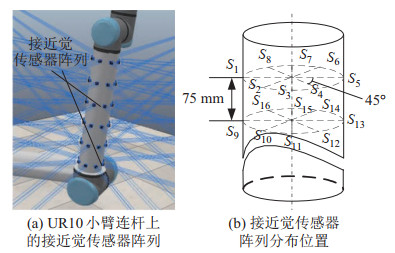图 1 接近觉传感器阵列分布 Fig.1 Distribution of proximity sensor array
2.2 障碍物表面建模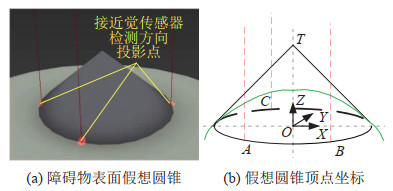图 2 障碍物局部表面建模 Fig.2 The modeling of obstacle local surface

$A $$B$$ C$点的空间坐标分别为$(x_{1}, y_{1}, z_{1}) $$(x_{2}, y_{2}, z_{2})$$ (x_{3}, y_{3}, z_{3})$，圆锥顶角为$\theta$，易求得$A $$B$$ C$三点的外接圆圆心$O$的坐标为$(x_{0}, y_{0}, z_{0})$、半径为$r$，显然可求得圆锥顶点$T$的坐标为

 \begin{align} \begin{bmatrix} {x_{T}} \\ {y_{ T}} \\ {z_{ T}} \end{bmatrix}=\mathit{\boldsymbol{v}}\cdot t+\begin{bmatrix} {x_{0}} \\ {y_{0}} \\ {z_{0}} \end{bmatrix} \end{align} (1)

 \begin{align} \mathit{\boldsymbol{v}} & =\begin{bmatrix} {x_{2} -x_{1}} \\ {y_{2} -y_{1}} \\ {z_{2} -z_{1}} \end{bmatrix} \begin{bmatrix} {x_{3} -x_{1}} \\ {y_{3} -y_{1}} \\ {z_{3} -z_{1}} \end{bmatrix} \end{align} (2)
 \begin{align} t & =\pm \frac{d^{2}}{ | \mathit{\boldsymbol{v}} | } \end{align} (3)
 \begin{align} d & =\frac{r}{ \tan \dfrac{\theta} {2}} \end{align} (4)

2.3 连杆运动方向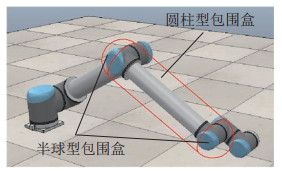图 3 UR10小臂包围盒 Fig.3 Bounding box of an UR10's forearm

 \begin{align} &U_{\rm{rep}} ({{\mathit{\boldsymbol{\varTheta}}}_{t}})=\\ &\begin{cases} \frac{1}{2}\eta \left({\frac{1}{\min({d_{\rm a} ({{\mathit{\boldsymbol{\varTheta}}}_{t} | \mathit{\boldsymbol{P}}}), d_{\rm b} ({{\mathit{\boldsymbol{\varTheta}}}_{t} | \mathit{\boldsymbol{P}}})})-r}-\frac{1}{d_{0}}}\right)^{2}, \\ \quad \quad 0<\min({d_{\rm a} ({{\mathit{\boldsymbol{\varTheta}}}_{t} | \mathit{\boldsymbol{P}}}), d_{\rm b} ({{\mathit{\boldsymbol{\varTheta}}}_{t} | \mathit{\boldsymbol{P}}})})-r\leqslant d_{0} \\ 0, \quad\rm{其他} \end{cases} \end{align} (6)

 \begin{align} U({{\mathit{\boldsymbol{\varTheta}}}_{t}^{*}})=\min\limits_{{\mathit{\boldsymbol{\varTheta}}}_{t} \in A_{{\mathit{\boldsymbol{\varTheta}}}}} \{{U({{\mathit{\boldsymbol{\varTheta}}}_{t}})}\} \end{align} (8)

3.2 基于绕行法的改进算法

 \begin{align} U({{\mathit{\boldsymbol{\varTheta}}}_{t}^{*}})=U({{\mathit{\boldsymbol{\varTheta}}}_{t-1}}) \end{align} (9)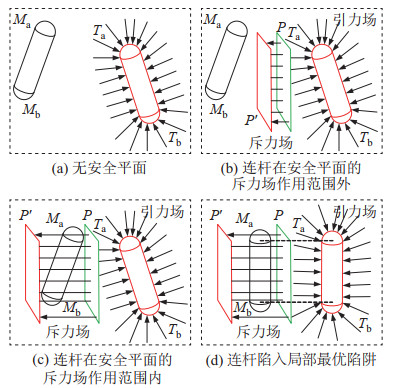图 7 人工势场法中连杆、安全平面与连杆目标位姿的关系 Fig.7 The relationship among link, safety plane and target pose of a link in artificial potential field method

 \begin{align} U_{\rm{att}} ({{\mathit{\boldsymbol{\varTheta}}}_{t}})=\;&\frac{1}{2}\zeta_{1} \sum\limits_ {i\ne m} ({\theta_{it} -\theta_{{\rm{goal}}\_i}})^{2} \pm \zeta_{2} \theta_{m_{t}} + \\ & k|{\mathit{\boldsymbol{\varTheta}}}_{t} -{\mathit{\boldsymbol{\varTheta}}}_{t-1} | \end{align} (10)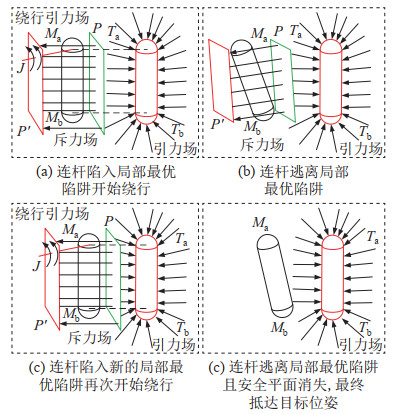图 8 绕行法逃离局部最优陷阱 Fig.8 Escaping the local optimum trap by the detour method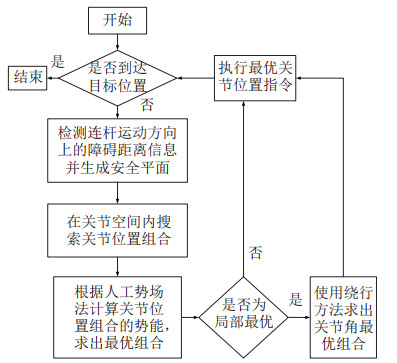图 9 算法流程图 Fig.9 Algorithm flow chart
4 实验（Experiment） 4.1 实验设置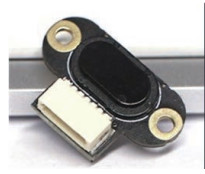图 10 实验用TOF传感器 Fig.10 The TOF sensor used in the experiment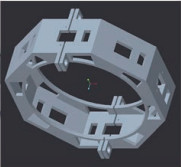图 11 传感器固定壳体 Fig.11 Sensor fixing housing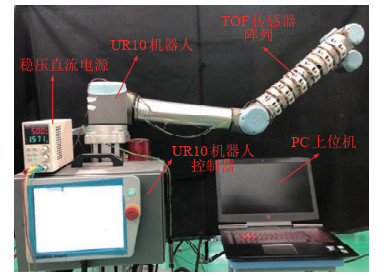图 12 实验控制系统 Fig.12 Control system in the experiment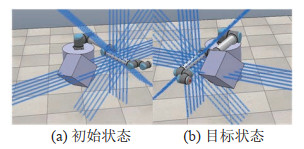图 13 UR10机器人设置示意图 Fig.13 Setting of the UR10 robot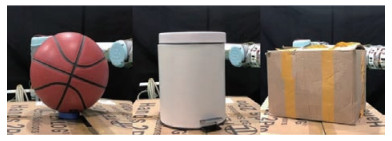图 14 球体、圆柱体、长方体障碍物 Fig.14 Spherical, cylindrical and rectangular obstacles
4.2 避障实验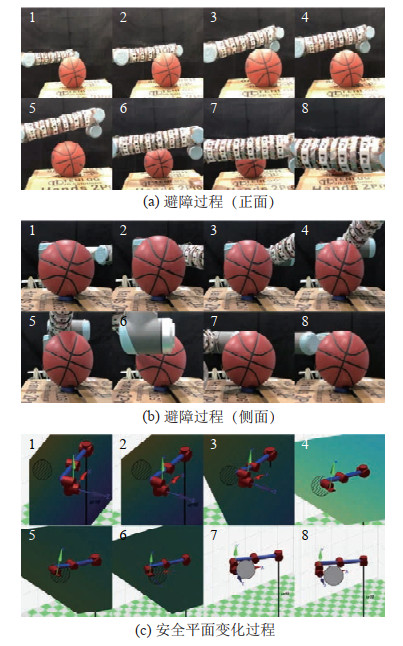图 15 球体障碍物避障实验 Fig.15 The experiment of avoiding spherical obstacle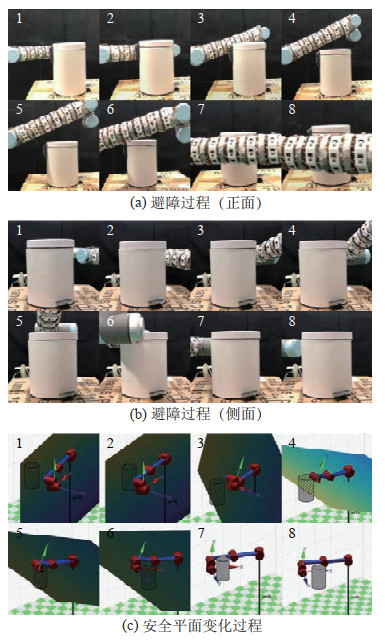图 16 圆柱体障碍物避障实验 Fig.16 The experiment of avoiding cylindrical obstacle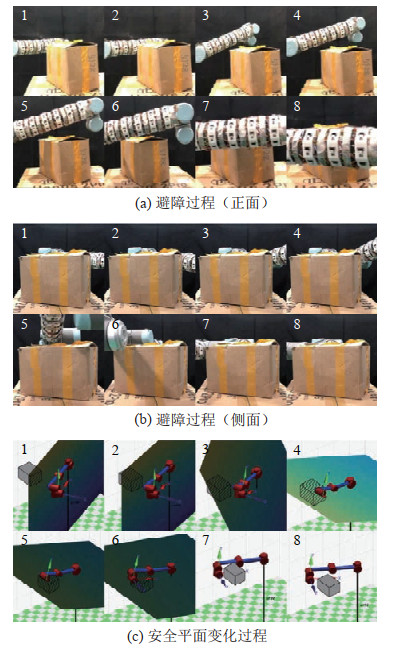图 17 长方体障碍物避障实验 Fig.17 The experiment of avoiding rectangular obstacle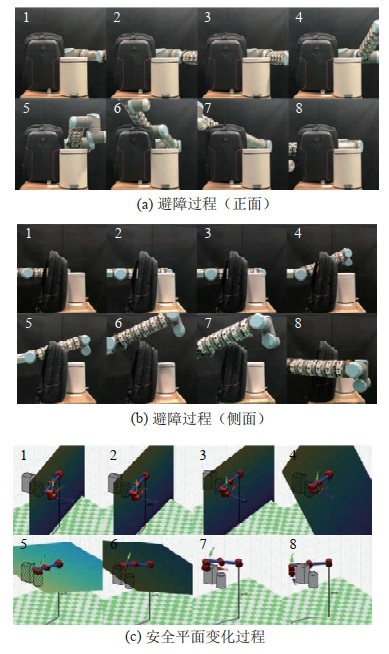图 18 背包与圆柱体障碍物避障实验 Fig.18 The experiment of avoiding bag and cylindrical obstacle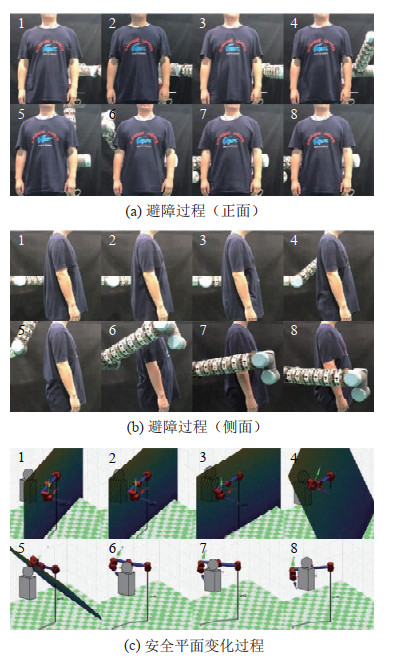图 19 人体躯干避障实验 Fig.19 The experiment of avoiding human body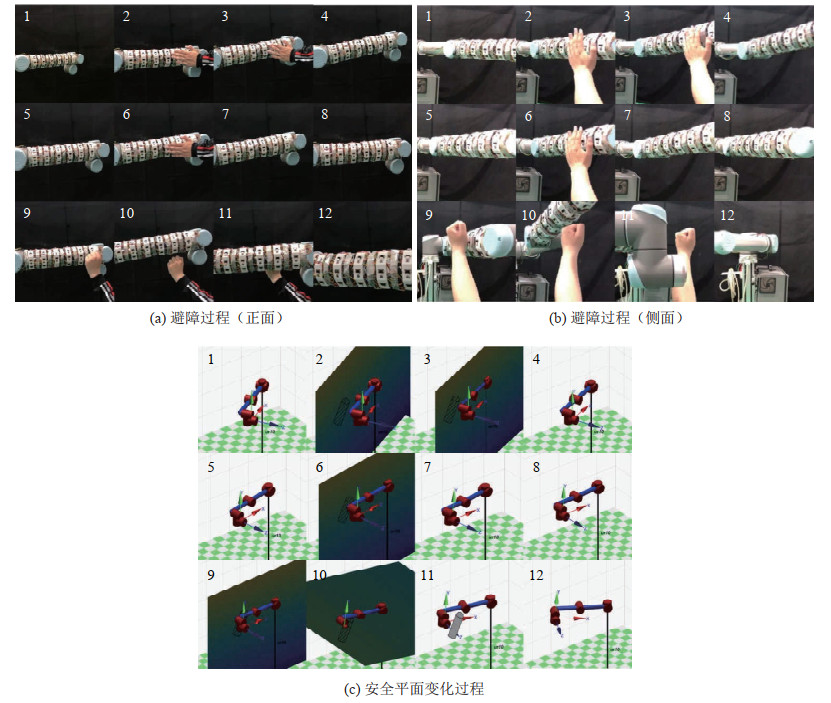图 20 手部干涉避障实验 Fig.20 The experiment of avoiding hand interference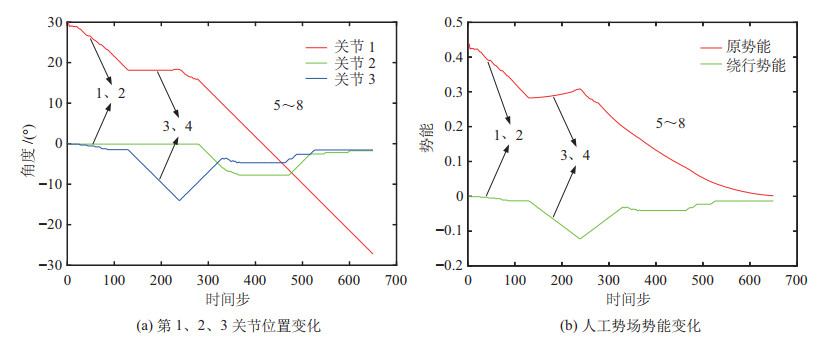图 21 球体障碍物避障实验数据 Fig.21 The experiment data of avoiding spherical obstacle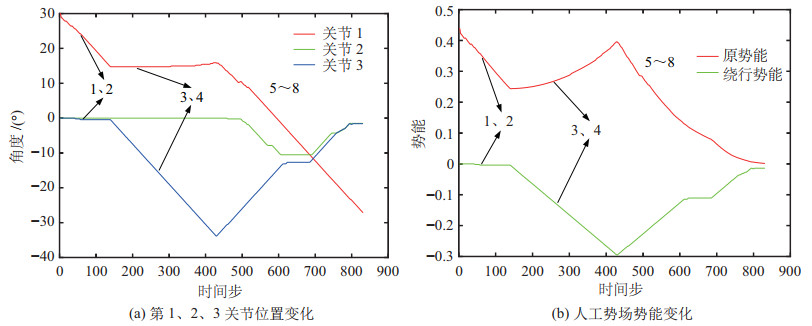图 22 圆柱体障碍物避障实验数据 Fig.22 The experiment data of avoiding cylindrical obstacle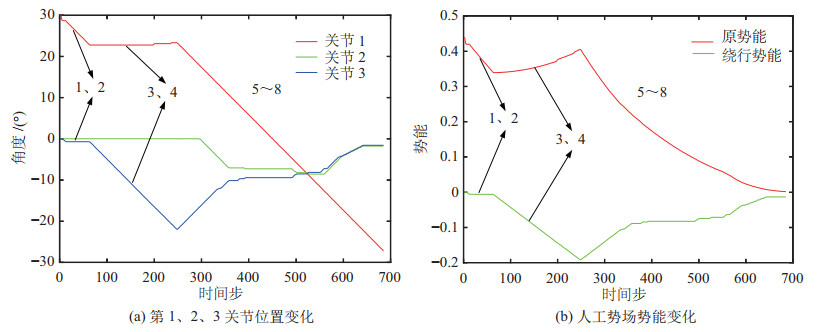图 23 长方体障碍物避障实验数据 Fig.23 The experiment data of avoiding rectangular obstacle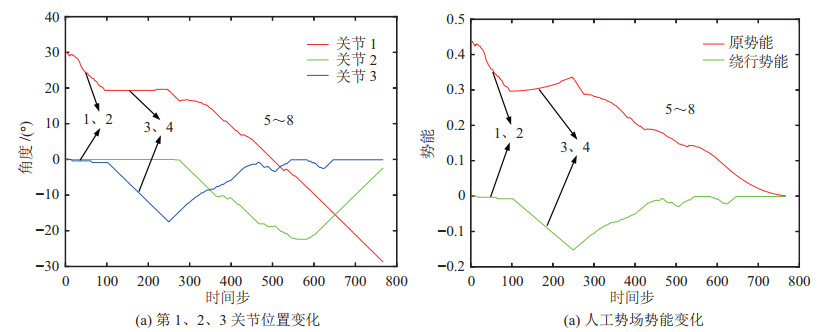图 24 背包与圆柱体障碍物避障实验数据 Fig.24 The experiment data of avoiding bag and cylindrical obstacle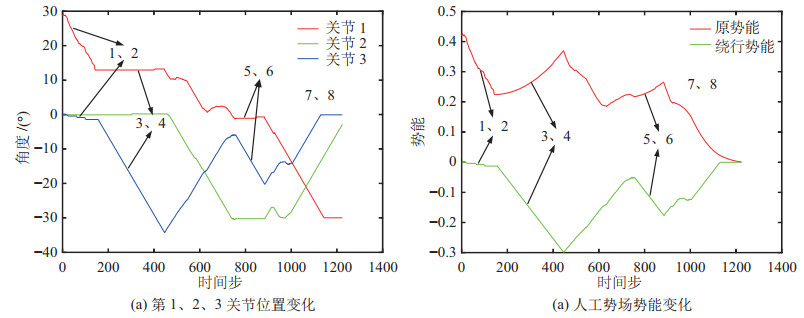图 25 人体躯干避障实验数据 Fig.25 The experiment data of avoiding human body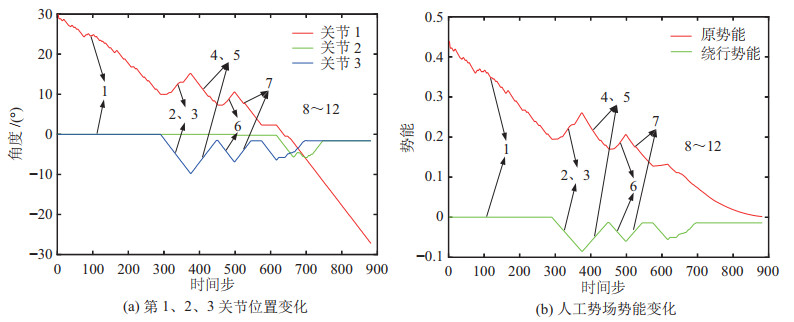图 26 手部干涉避障实验数据 Fig.26 The experiment data of avoiding hand interference

5 结论（Conclusion）

  Zhao X L, Zhang J, Tian J M, et al. Multiscale object detection in high-resolution remote sensing images via rotation invariant deep features driven by channel attention[J]. International Journal of Remote Sensing, 2021, 42(15): 5764-5783. DOI:10.1080/01431161.2021.1931537  Giveki D. Robust moving object detection based on fusing Atanassov's intuitionistic 3D fuzzy histon roughness index and texture features[J]. International Journal of Approximate Reasoning, 2021, 135: 1-20. DOI:10.1016/j.ijar.2021.04.007  Ren S Q, He K M, Girshick R, et al. Faster R-CNN: Towards real-time object detection with region proposal networks[J]. IEEE Transactions on Pattern Analysis and Machine Intelligence, 2017, 39(6): 1137-1149. DOI:10.1109/TPAMI.2016.2577031  郭毓, 苏鹏飞, 吴益飞, 等. 基于Faster R-CNN的机器人目标检测及空间定位[J]. 华中科技大学学报(自然科学版), 2018, 46(12): 55-59. Guo Y, Su P F, Wu Y F, et al. Object detection and location of robot based on Faster R-CNN[J]. Journal of Huazhong University of Science and Technology (Natural Science Edition), 2018, 46(12): 55-59.  杜学丹, 蔡莹皓, 鲁涛, 等. 一种基于深度学习的机械臂抓取方法[J]. 机器人, 2017, 39(6): 820-828, 837. Du X D, Cai Y H, Lu T, et al. A robotic grasping method based on deep learning[J]. Robot, 2017, 39(6): 820-828, 837. DOI:10.13973/j.cnki.robot.2017.0820  Chao Y, Chen X C, Xiao N F. Deep learning-based grasp-detection method for a five-fingered industrial robot hand[J]. IET Computer Vision, 2019, 13(1): 61-70. DOI:10.1049/iet-cvi.2018.5002  Schwarz M, Milan A, Periyasamy A S, et al. RGB-D object detection and semantic segmentation for autonomous manipulation in clutter[J]. International Journal of Robotics Research, 2017, 37(4-5): 437-451.  任亚冰. 基于机器学习的机械臂运动规划研究[D]. 成都: 电子科技大学, 2019. Ren Y B. Research on trajectory planning of manipulator based on machine learning[D]. Chengdu: University of Electronic Science and Technology of China, 2019.  Tsuji S, Kohama T. Proximity and contact sensor for human cooperative robot by combining time-of-flight and self-capacitance sensors[J]. IEEE Sensors Journal, 2020, 20(10): 5519-5526. DOI:10.1109/JSEN.2020.2969653  宗成星, 陆亮, 雷新宇, 等. 一种基于A*算法的空间多自由度机械臂路径规划方法[J]. 合肥工业大学学报(自然科学版), 2017, 40(2): 164-168. Zong C X, Lu L, Lei X Y, et al. A path planning approach for multi-DOF spatial manipulator via A* algorithm[J]. Journal of Hefei University of Technology (Natural Science), 2017, 40(2): 164-168. DOI:10.3969/j.issn.1003-5060.2017.02.005  王伟, 杨扬, 原魁, 等. 机器人C-空间障碍边界建模与无碰路径规划[J]. 机器人, 1998, 20(4): 280-286. Wang W, Yang Y, Yuan K, et al. Robot C-obstacle boundary modeling and collision-free path planning[J]. Robot, 1998, 20(4): 280-286. DOI:10.3321/j.issn:1002-0446.1998.04.007  刘晓磊, 蒋林, 金祖飞, 等. 非结构化环境中基于栅格法环境建模的移动机器人路径规划[J]. 机床与液压, 2016, 44(17): 1-7. Liu X L, Jiang L, Jin Z F, et al. Mobile robot path planning based on environment modeling of grid method in unstructured environment[J]. Machine Tool and Hydraulics, 2016, 44(17): 1-7.  李洋, 徐达. 基于引力自适应步长RRT的双臂机器人协同路径规划[J]. 机器人, 2020, 42(5): 606-616. Li Y, Xu D. Cooperative path planning of dual-arm robot based on attractive force self-adaptive step size RRT[J]. Robot, 2020, 42(5): 606-616.  关英姿, 宋春林, 董惠娟. 空间自由漂浮机器人对运动目标抓捕的路径规划[J]. 机器人, 2017, 39(6): 803-811. Guan Y Z, Song C L, Dong H J. Path planning of the free-floating manipulator for capturing a moving target[J]. Robot, 2017, 39(6): 803-811.  李东方, 李科伟, 邓宏彬, 等. 基于人工势场与IB-LBM的机器蛇水中2D避障控制算法[J]. 机器人, 2018, 40(3): 346-359. Li D F, Li K W, Deng H B, et al. The 2D aquatic obstacle avoidance control algorithm of the snake-like robot based on artificial potential field and IB-LBM[J]. Robot, 2018, 40(3): 346-359.  李晔, 姜言清, 张国成, 等. 考虑几何约束的AUV回收路径规划[J]. 机器人, 2015, 37(4): 478-485. Li Y, Jiang Y Q, Zhang G C, et al. AUV recovery path planning method considering geometrical constraints[J]. Robot, 2015, 37(4): 478-485.  Zhang Y T, Zhao G. Research on multi-service demand path planning based on continuous Hopfield neural network[M]// Lecture Notes in Electrical Engineering, Vol. 286. Berlin, Germany: Springer, 2014: 417-430.  李娜. 面向人机协作安全保障的工业机器人路径规划研究与实现[D]. 武汉: 武汉理工大学, 2018. Li N. Research and implementation on path planning of industrial robot towards safety assurance of human-robot collaboration[D]. Wuhan: Wuhan University of Technology, 2018.  汪首坤, 朱磊, 王军政. 基于导航势函数法的六自由度机械臂避障路径规划[J]. 北京理工大学学报, 2015, 35(2): 186-191. Wang S K, Zhu L, Wang J Z. Path plan of 6-DOF robot manipulators in obstacle environment based on navigation potential function[J]. Transactions of Beijing Institute of Technology, 2015, 35(2): 186-191.  Sang H Q, You Y S, Sun X J, et al. The hybrid path planning algorithm based on improved A* and artificial potential field for unmanned surface vehicle formations[J]. Ocean Engineering, 2021, 223. DOI:10.1016/j.oceaneng.2021.108709  宋建辉, 代涛, 刘砚菊, 等. 基于改进人工势场法的移动机器人路径规划[J]. 计算机工程与科学, 2017, 39(7): 1328-1332. Song J H, Dai T, Liu Y J, et al. Path planning of mobile robots based on improved artificial potential field method[J]. Computer Engineering and Science, 2017, 39(7): 1328-1332.  周自维, 周冰, 赵雪. 基于改进人工势场的多机器人编队控制[J]. 科技创新与应用, 2015(33): 44-45. Zhou Z W, Zhou B, Zhao X. Formation control of multi robots with improved artificial potential field method[J]. Technology Innovation and Application, 2015(33): 44-45.